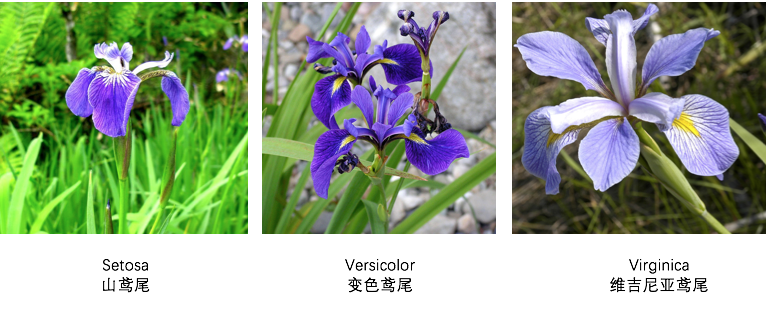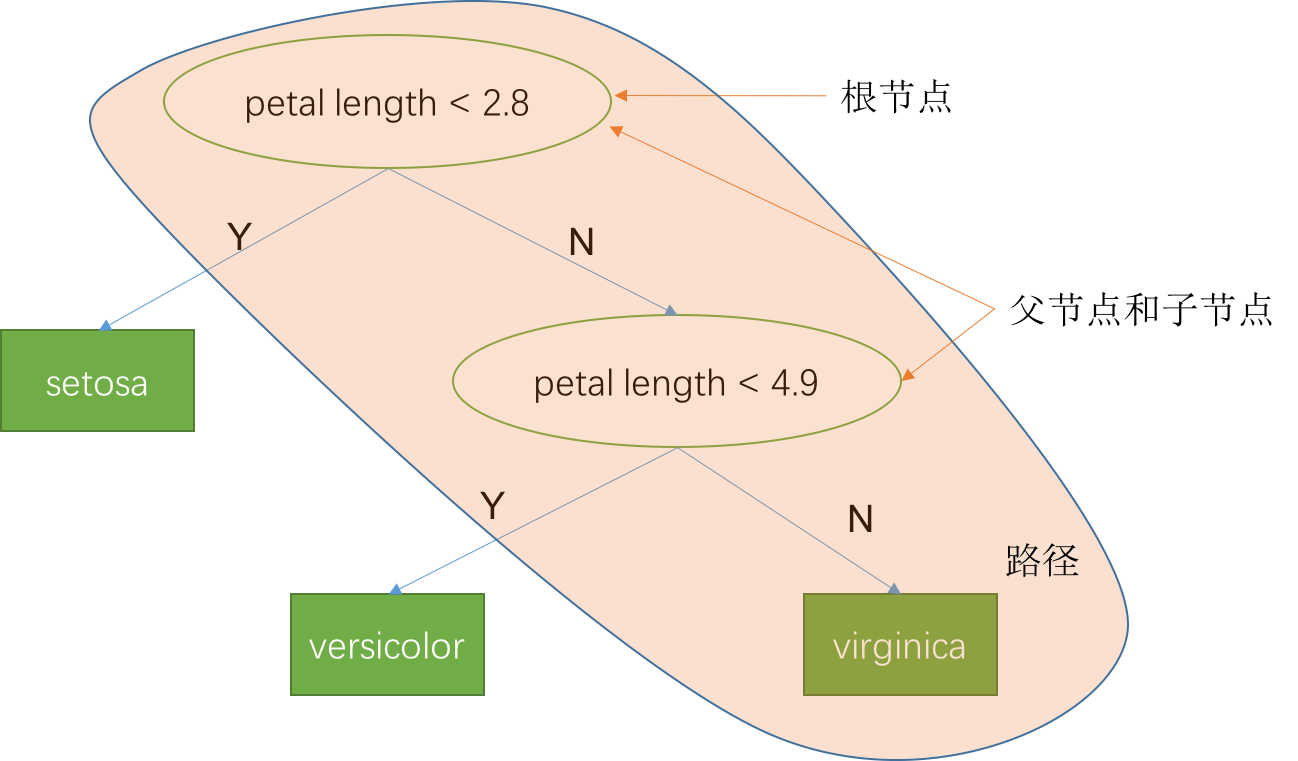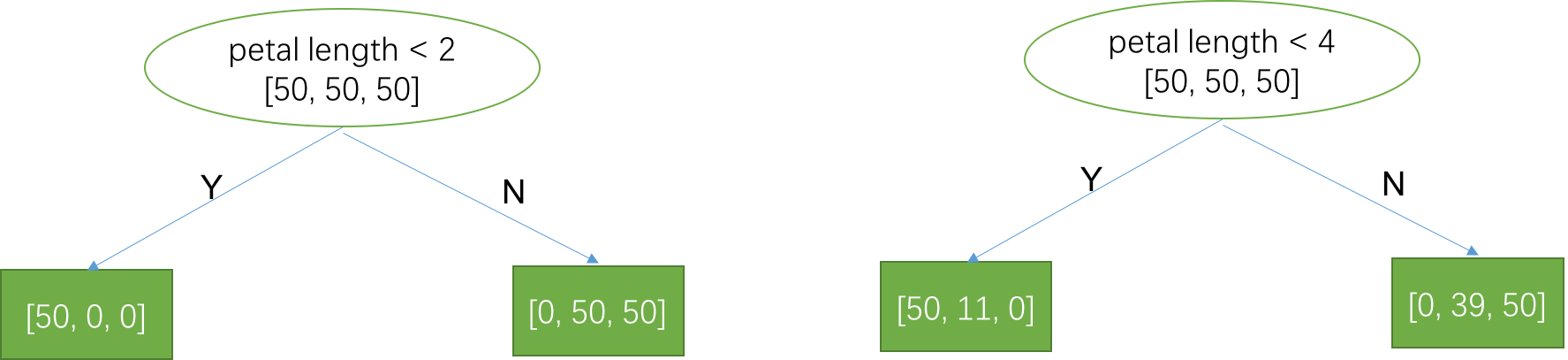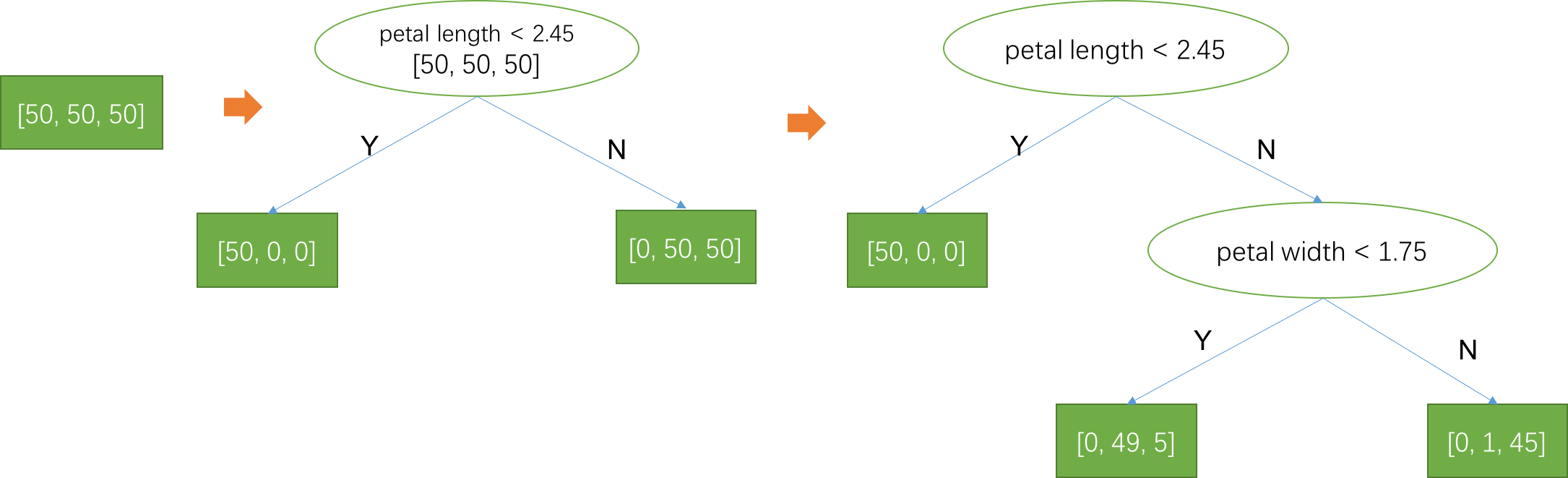## 关于

1. 什么是决策树模型？
2. 构建决策树模型的算法是怎么实现的？

1. 至少有高中数学水平。
2. 如果你需要完成实践部分，需要有基本的 python 知识，你可以通过python快速入门快速了解python如何使用。

sepal length (cm) sepal width (cm) petal length (cm) petal width (cm) target
91 6.1 3.0 4.6 1.4 1
77 6.7 3.0 5.0 1.7 1
99 5.7 2.8 4.1 1.3 1
65 6.7 3.1 4.4 1.4 1
14 5.8 4.0 1.2 0.2 0
108 6.7 2.5 5.8 1.8 2
142 5.8 2.7 5.1 1.9 2
127 6.1 3.0 4.9 1.8 2
24 4.8 3.4 1.9 0.2 0
2 4.7 3.2 1.3 0.2 0

• sepal length (cm) 萼片长度
• sepal width (cm) 萼片宽度
• petal length (cm) 花瓣长度
• petal width (cm) 花瓣宽度

• 0： setosa 山鸢尾
• 1： versicolor 变色鸢尾
• 2： virginica 维吉尼亚鸢尾## 回顾决策树1. IF 花瓣长度(petal length) < 2.8, THEN sentosa
2. IF 花瓣长度(petal length) >= 2.8 AND 花瓣长度(petal length) < 4.9, THEN versicolor
3. IF 花瓣长度(petal length) >= 4.9, THEN virginica

$$y = \begin{cases} 0, \text{petal length} \lt 2.8 \\ 1, \text{petal length} \in [2.8, 4.9) \\ 2, \text{petal length} \ge 4.9 \end{cases}$$

• 子节点：和节点相连的后继节点，比如节点(petal length < 4.9)是节点(petal length < 2.8)的子节点
• 父节点：当前节点是子节点的父节点。比如节点(petal length < 2.8)是节点(petal length < 4.9)的父节点
• 边：相连相邻两个节点中间的部分叫边，这条边有方向，从父节点指向子节点。
• 中间节点：有子节点的节点，它不直接输出预测结果的节点，对应一个规则。图中椭圆形的节点都是中间节点。
• 叶子节点：没有子节点的节点，直接输出预测结果的节点，对应一个复杂的规则，通常是多个规则的组合。图中方形的节点都是叶子节点。
• 根节点：没有父节点的中间节点。节点(petal length < 2.8)就是上述决策树的根节点。
• 路径：从根节点出发，沿着子节点移动，到达叶子节点时所经历的所有节点序列就是一条路径，图中黄色区域表示出一条路径。路径上边的数量叫做路径长度，实际上也等于中间节点的数目。
• 深度：最大的路径长度，比如上述决策树深度为2.
• 规则：一个可以判定真假的表达式就叫规则，比如花瓣长度(petal length) < 2.8
• 组合规则：多个规则通过且(AND)和或(OR)连接起来的语句叫做组合规则，比如 花瓣长度(petal length) >= 2.8 AND 花瓣长度(petal length) < 4.9

## 决策树生成算法### 信息熵

$$H(\{p_i\}) = -\sum_i p_i \log p_i$$#### 平均信息量解释

$$-\sum_i p_i \log p_i = -\sum \frac{1}{6} \log \frac{1}{6} = \log 6$$

### 信息增益准则

$$\Delta I = \text{分裂前的不确定度} - \text{分列后的不确定度} \\ = H(P) - [r_1 H(C_1) + r_2 H(C_2)]$$

P是分裂前数据集中每一类的概率分布，在上述的第一种分裂中，分裂前三个类别的样本都是50，所以每一类都是1/3的概率，所以$H(P) = \log 3 = 1.098$。C1是分裂后分到左子树的数据中每一类的概率分布，在分裂规则petal length<2下，分到左子树的样本有50个，且全部是第一类，所以三个类别的概率分布是$\{1, 0, 0 \}$，$H(C_1) = 1\log1 + 0\log 0 + 0\log0 = 0(0 \log 0 = 0)$。$r_1$是左子树的样本占分裂前的比例，$r_1 = 50/150=1/3$。同样，分到右子树的样本分别是[0, 50, 50]，所以$H(C_2) = 2/3\log 2 = 0.462, r_2=2/3$。因为分裂后有两个样本集合，所以需要把这两个集合的不确定度平均一下，平均的方式是按照样本数目的加权平均。根据信息增益公式，可以得到 $\Delta I = 1.098 - [1/3 * \log 0 + 0.462*2/3] = 0.79$。

1. 计算分裂前的信息熵H(P)
2. 对每一个特征的每一个可能的分裂点，计算分裂后的信息增益，找到最大增益的特征$x_i$和分裂点$t_i$
3. 得到分裂规则 $x_i < t_i$ 和最大信息增益

1. 初始化A=训练集
2. 如果集合A的数据量小于一个阈值或者只有一类样本，停止分裂。否则进入第3步。
3. 对集合A，依次选取第i个特征进行分裂，利用分裂算法计算该特征的信息增益和分裂规则，选取信息增益最大的特征作为当前分裂特征，对应的分裂规则作为当前的分裂规则。
4. 利用第3步生成的分裂规则，将集合A划分为两个子集B和C。对这两个子集继续应用生成算法生成左子树和右子树。## 决策树的几何解释## 思考与实践

1. 证明对于任何一种分裂方式，信息增益非负。
2. 实现上述基于信息增益的决策树生成算法，启动代码已提供
from sklearn.datasets import load_iris
import numpy as np
from scipy import stats

def H(arr):
"""计算熵的函数"""
n = sum(arr)
arr = [1.0*i/n for i in arr]
return sum([- p * np.log(p) for p in arr if p >0])

def InformactionInc(x, y, split):
"""计算利用split分裂特征x时，信息增益
x : np.array 特征数组, size= (样本数, )
y : np.array 类别， size=(样本数, )
split : double 候选分裂点的值

return : 信息增益
"""
delta = 0

# end

return delta

def FindSpliter(X, y):
"""寻找最佳分裂特征和分裂点算法
X : np.array 特征，size = (样本数, 特征数)
y : np.array 类别，size = (样本数, )
return (分裂特征索引 i, 分裂点的值 t)
"""
n_samples , n_features = X.shape

max_infor_inc = 0
best_feature = 0
best_split_value = None

# end

return best_feature, best_split_value

def TreeGenerate(X, y, depth=0, max_depth=3):
"""决策树生成算法，返回一棵树
"""
# 满足不分裂准则
if X.shape < 10 or np.unique(y).shape == 1 or depth >= max_depth:
node = {'prediction' : stats.mode(y)}
return node

# 继续分裂
best_feature, best_split_value = FindSpliter(X, y)
mask = X[:, best_feature] < best_split_value
node = {
}
return node

def TreePredict(X, T):
"""实现决策树预测算法"""
pass